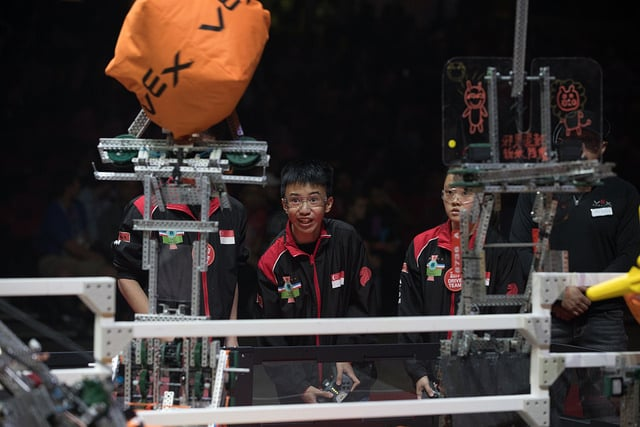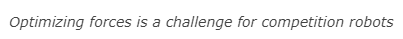STEM Labs
Momentum Alley Teacher

Teacher Portal### How Physics can Affect Competition Robots

When designing a robot for VEX Robotics Competitions, you must remember that any motor will be fighting against the robot's inertia whenever the robot is running. Inertia is an object's resistance to changes in its velocity. Inertia is increased as the object's mass and therefore its momentum are increased. This means if you add mass to your robot and make it heavier than it has to be, the motors will not be as effective in changing the robot's velocity! Therefore, you should try to use as light and as few materials as possible if you want to maximize the motors' efficiencies.

On the other hand, running a light robot very quickly can also lead to difficulties. If you are trying to make precise and accurate movements in the course of a competition, you may need to ease off of the power by reducing the velocity during your movements.

Let's explore the idea that the momentum of two colliding objects predicts what will happen after they collide. This is an important factor to consider when developing competition projects because you want your robot to move as fast as possible. You also want to have as many components as possible built into the robot that will give it an advantage for manipulating and collecting during the game.

Momentum is the amount of motion that an object has and is determined by the moving object's mass and velocity. So, a competition robot with all of its components can be heavy and moving as fast as possible. Therefore, its momentum is very high. This is when you need to consider what happens when it comes into contact with parts of the field or other robots.

Look back at your table from the Exploring Velocity activity. You tested the transfer of energy during collisions by setting different velocities for the robot and driving it forward until it hit the ball. You should have noticed that higher velocities set for the robot pushed the ball farther after a collision than lower velocities did. This is an obvious effect of the robot's momentum because the mass of the robot remained the same but the velocity increased and therefore, its momentum increased.

Something important to consider about that test is that the ball was not moving. It had a velocity, a momentum, and an acceleration of all zeros before the robot collided with it. Importantly, its mass was likely far less than the robot's mass. After the collision, its acceleration and therefore its velocity and its momentum all increased. How fast the ball's velocity was after the collision depended in part on the mass of the ball. Lighter balls accelerate and move faster. If your class used a ball with more mass, imagine a bowling ball, the ball might have moved slowly and not very far after the collision.

Again, this is important to consider when planning for a competition because you can break parts of the field, parts of your robot, or parts of other robots if the robot's momentum is too high. Imagine if your robot had a high velocity and crashed into an object that couldn't roll away like the ball in the previous activity. That object could have been broken by the impact forces (energy) of the collision.Teacher Toolbox - Explaining a Collision Further

Another important point in understanding the influence of velocity on momentum is the difference between acceleration and velocity. Acceleration is the rate of change in velocity. In the previous Exploring Velocity activity, acceleration was an important factor because the ball was at rest prior to the collision. So it is due to the acceleration caused by the collision that the ball then reaches its final velocity.
This connects to Newton's Second Law of Motion: that the acceleration of an object is dependent upon two variables - the net or sum of the force(s) acting on the object, and the mass of the object. The net force acting on the ball was arguably zero because it was at rest. Its acceleration after being hit by the robot was the product of the force (momentum) of the robot and the amount of mass of the ball. Heavier balls (e.g., a bowling ball or basketball) in this activity would not have accelerated as fast as lighter balls (e.g., a soccer ball or a bouncy inflatable ball).Extend Your Learning - Baseball

To relate this activity to other collisions, students can investigate the speed of a baseball batter's swing and how fast baseballs travel after colliding with or being struck by the bat. Have them consider the mass of the bat and the trade-offs between the bat's mass (wooden vs aluminum), its speed when swung, and its momentum as it strikes the ball.